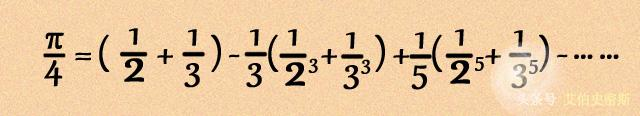A-A+

# 圆周率算法

2009年04月20日 阅读 1 次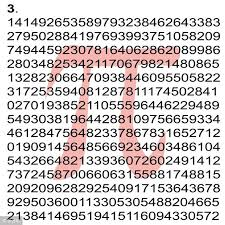```#include < stdio.h >
long  a = 10000 ,b = 0 ,c = 2800 ,d,e,f[ 2801 ],g;
void  main()
{
printf( " %d " ,b);
for (;b != c;)
{
f[b] = a / 5 ;
b ++ ;
}
for (; d = 0 ,g = c * 2 ; c -= 14 ,printf( " %.4d " ,e + d / a),e = d % a)
for (b = c;d += f[b] * a,f[b] = d %-- g,d /= g -- , -- b;d *= b);
}```

先不管它,这个程序虽然风格恶劣(“短小精悍”让人看不懂),但这个算法还是值得研究的.毕竟,它是确定性算法,比各种随机版本(比如蒙特卡罗法模拟)的算法要可靠.

```int  a = 10000 ,b,c = 2800 ,d,e,f[ 2801 ],g;
main(){for (;b - c;)f[b ++ ] = a / 5 ;for (;d = 0 ,g = c * 2 ;c  -= 14 ,printf( " %.4d " ,e + d / a),e = d % a)for (b = c; d += f[b] * a,f[b] = d %-- g,d /= g -- , -- b; d *= b);}```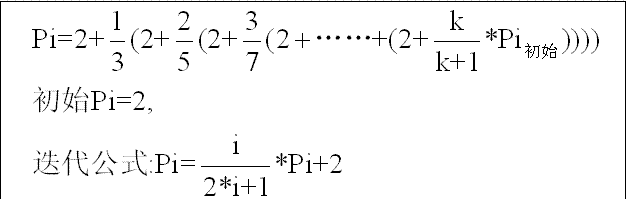#include "stdio.h"
void main()
{
float pi=2;
int  i;
for(i=100;i>=1;i--)
pi=pi*(float)i/(2*i+1)+2;
printf("%fn",pi);
getchar();
}

```int  a = 10000 ,b,c = 2800 ,d,e,f[ 2801 ],g;
main(){
int  i;
for (i = 0 ;i < c;i ++ )
f[i] = a / 5 ;
while (c != 0 )
{
d = 0 ;
g = c * 2 ;
b = c;
while ( 1 )
{
d = d + f[b] * a;
g -- ;
f[b] = d % g;
d = d / g;
g -- ;
b -- ;
if (b == 0 )  break ;
d = d * b;
}
c = c - 14 ;
printf( " %.4d " ,e + d / a);
e = d % a;
}
}```

int a=10000,b,c=2800,d,e,f,g;

c的大小随便改,举个例子: 比如,
a=100000,c=c-14,迭代2800次,共循环200次,输出1000位
a=1000,c=c-7,迭代2800次,共循环400次,输出1200位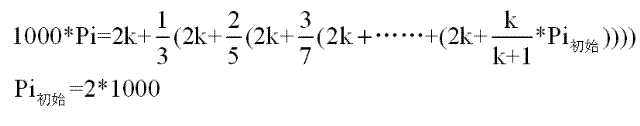```for (i = 0 ;i < c;i ++ )f[i] = a / 5 ;你可能会觉得奇怪，为什么这里要把一个常数储存到数组中去，请继续往下看。我们先来跟踪一下程序的运行：while (c != 0 )  假设这是第一次运行，c = 2800 ，为迭代次数
{
d = 0 ;
g = c * 2 ;  这里的g是用来做k / (2k + 1 )中的分母
b = c;    这里的b是用来做k / (2k + 1 )中的分子
while ( 1 )
while ( 1 )
{
d = d + f[b] * a; f中的所有的值都为2000，这里在计算时又把系数扩大了a = 10000倍。这样做的目的稍候介绍，你可以看到输出的时候是d / a,所以这不影响计算.
g -- ;
f[b] = d % g; 先不管这一行
d = d / g;   第一次运行的g为2 * 2799 + 1 ，你可以看到g做了分母
g --```

;
b -- ;
if (b == 0 )  break ;
d = d * b; 这里的b为2799，可以看到d做了分子。
}
c = c - 14 ;
printf( " %.4d " ,e + d / a);
e = d % a;
}d=d/g, 这一行的目的是除以2k+1，我们知道之所以程序无法精确计算的原因就是这个除法。即使用浮点数，答案也是不够精确的，因此直接用来计算800位的Pi是不可能的。那么不精确的成分在哪里？很明显：就是那个余数d%g。程序用f数组把这个误差储存起来，再下次计算的时候使用。现在你也应该知道为什么 d=d+f[b]*a;中间需要乘上a了吧。

d如果不乘10000这个系数，则其值为2000，那么运行d=d/g；则是2000/(2*2799+1)，这种整数的除法答案为0，根本无法迭代下去了。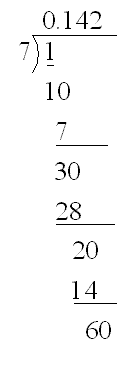```for (i = 0 ;i < 800 ;i ++ )
{
{
d = 0 ;
g = c * 2 ;
b = c;
while ( 1 )
{
d = d + f[b] * a;
g -- ;
f[b] = d % g;
d = d / g;
g -- ;
b -- ;
if (b == 0 )  break ;
d = d * b;
}
//  c=c-14; 不要这句话。
printf( " %.4d " ,e + d / a);
e = d % a;
}

```

```1415926535 8979323846 2643383279 5028841971 6939937510
5820974944 5923078164 0628620899 8628034825 3421170679
8214808651 3282306647 0938446095 5058223172 5359408128
4811174502 8410270193 8521105559 6446229489 5493038196
4428810975 6659334461 2847564823 3786783165 2712019091
4564856692 3460348610 4543266482 1339360726 0249141273
7245870066 0631558817 4881520920 9628292540 9171536436
7892590360 0113305305 4882046652 1384146951 9415116094
3305727036 5759591953 0921861173 8193261179 3105118548
0744623799 6274956735 1885752724 8912279381 8301194912
9833673362 4406566430 8602139494 6395224737 1907021798
6094370277 0539217176 2931767523 8467481846 7669405132
0005681271 4526356082 7785771342 7577896091 7363717872
1468440901 2249534301 4654958537 1050792279 6892589235
4201995611 2129021960 8640344181 5981362977 4771309960
5187072113 4999999837 2978049951 0597317328 1609631859
5024459455 3469083026 4252230825 3344685035 2619311881
7101000313 7838752886 5875332083 8142061717 7669147303
5982534904 2875546873 1159562863 8823537875 9375195778
1857780532 1712268066 1300192787 6611195909 2164201989```